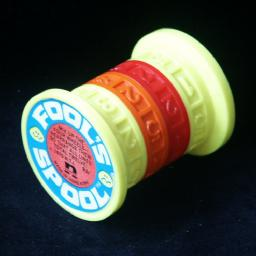# Fool's SpoolFool's Spool looks like a large thread spool, i.e. it is a cylinder with a rim at both ends. It consists of 4 disks which can rotate. Each disk has 8 numbers around it ranging from 1 to 5. The aim of the puzzle is to rotate the disks so that the 8 lines of four numbers each sum up to 12.

The label on the puzzle states that it was made in 1970 by Norstar (N.T.I.). The numbers on the four disks are:
15423435
12233543
12315243
14323543

## The number of positions:

If you hold the first disk steady, then the other three disks each have 8 positions relative to the first. This gives 83=512 positions.

## Solution:

The number of positions is relatively small, so it is quite easy to search through all of them by hand. For each of the eight positions of the second disk, there will be a pair that sums to 2, 3, 9 or 10. That very much limits the possible positions of the other two disks.
It is an interesting fact that there are exactly eight possible ways to sum to 12 with numbers in the range 1 to 5. In the unique solution, all eight ways occur. This is clearly an intentional part of the design. The solution is as follows:

 5 1 1 5 1 2 5 4 5 2 2 3 4 3 4 1 2 3 3 4 3 5 1 3 4 4 2 2 3 3 3 3